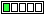All about flooble | fun stuff | Get a free chatterbox | Free JavaScript | Avatarsperplexus dot infoGetting 189 (Posted on 2016-09-05)Evaluate cubes of three consecutive positive integers.
Add up the digits in each of the three results, and add again until you’ve reached a single digit for each of the three numbers.
For example:
46^3 = 97336; 9 + 7 + 3 + 3 + 6 = 28; 2 + 8 = 10; 1 + 0 = 1
47^3 = 103823; 1 + 0 + 3 + 8 + 2 + 3 = 17; 1 + 7 = 8
48^3 = 110592; 1 + 1 + 0 + 5 + 9 + 2 = 18; 1 + 8 = 9

Putting the three digits in ascending order will always give the result 189.

Source:Kendall and Thomas "Mathematical Puzzles for the Connoisseur (1971)"

 No Solution Yet Submitted by Ady TZIDON No RatingSubject Author Datesolution Charlie 2016-09-05 10:05:38Please log in:

 Search: Search body:
Forums (0)